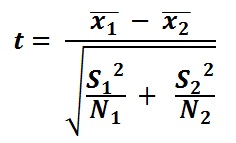## T Test Calculator

 Result: Mean of First Set Mean of Second Set SD of First Set SD of Second Set T Test Value

The T Test Calculator to calculate the significance of observed differences between the means of two samples.

### T Test formulawhere

2 is the mean of first data set

1 is the mean of first data set

S22 is the standard deviation of first data set

S12 is the standard deviation of first data set

N2 is the number of elements in the first data set

N1 is the number of elements in the first data set

Thinkcalculator.com provides you helpful and handy calculator resources.